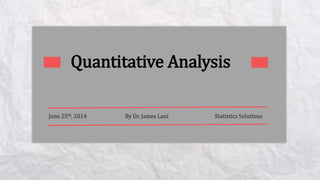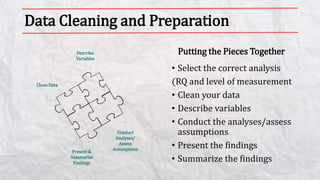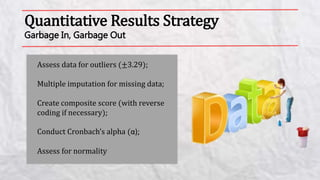Successfully reported this slideshow.

# Quantitative Analysis: Conducting, Interpreting, & Writing×

# Quantitative Analysis: Conducting, Interpreting, & Writing

In this webinar Dr. Lani discusses key points in successfully completing your quantitative analysis. You will learn how to conduct common statistical analyses, how to examine assumptions, how to easily generate APA 6th edition tables and figures, how to use Intellectus Statistics(TM) Software, how to identify and interpret the appropriate statistics, and how to present and summarize your findings.

SSP is now Intellectus Statistics Software. Intellectus Statistics™ software primarily serves the academic and research communities as a powerful statistical package that can be purchased via four distinct cloud based subscriptions. Learn more here: http://www.statisticssolutions.com/buy-intellectus/

In this webinar Dr. Lani discusses key points in successfully completing your quantitative analysis. You will learn how to conduct common statistical analyses, how to examine assumptions, how to easily generate APA 6th edition tables and figures, how to use Intellectus Statistics(TM) Software, how to identify and interpret the appropriate statistics, and how to present and summarize your findings.

SSP is now Intellectus Statistics Software. Intellectus Statistics™ software primarily serves the academic and research communities as a powerful statistical package that can be purchased via four distinct cloud based subscriptions. Learn more here: http://www.statisticssolutions.com/buy-intellectus/

### Quantitative Analysis: Conducting, Interpreting, & Writing

1. 1. Quantitative Analysis June 25th, 2014 By Dr. James Lani Statistics Solutions
2. 2. Data Cleaning and Preparation • Select the correct analysis (RQ and level of measurement • Clean your data • Describe variables • Conduct the analyses/assess assumptions • Present the findings • Summarize the findings Putting the Pieces TogetherDescribe Variables Clean Data Present & Summarize Findings Conduct Analyses/ Assess Assumptions
3. 3. Quantitative Results Strategy Garbage In, Garbage Out Assess data for outliers (±3.29); Multiple imputation for missing data; Create composite score (with reverse coding if necessary); Conduct Cronbach’s alpha (α); Assess for normality
4. 4. Descriptive Statistics Means & Standard Deviations, Frequency & Percentages Variable n % Location Urban 72 48.0 Rural 78 52.0 Ethnicity White 36 24.0 Hispanic 13 28.7 Other 71 47.3 Table 1 Frequencies and Percentages for Nominal Variables
5. 5. Chi-Square Goodness of Fit & Test of Independence Chi-square analysis answers what research questions? Assumptions of analysis: • Each cell has count of 1; • 80% of cells have an expected value of 5. Conducting analysis; Presenting findings; Write up in narrative; Tables and figures. Republican Democrat Green Independent Libertarian X X X X X Repub. Democrat Green Indep. Libert. Male X X X X X Female X X X X X
6. 6. Pearson Correlation Examines the relationship between two or more scales level variables Assumptions of the analysis: • Linearity • Homoscedacity • Normality Assumptions Linear Non-Linear Homoscedasticity Met Heteroscedasticity Normal Non-normal
7. 7. Independent Samples t-test Let’s look at differences in IQ by Gender Examines mean differences on a scale level dependent variable by a dichotomous nominal level independent variable. Assumptions of analysis: • Homogeneity of variance • Normality Males Females Part 1=1 Part 4=2 Part 2=2 Part 5=3 Part 3=3 Part 6=4 X=2 X=3 Males Females Part 1=1.9 Part 4=2.9 Part 2=2.0 Part 5=3.0 Part 3=2.1 Part 6=3.1 X=2 X=3
8. 8. One-Way ANOVA Let’s look at differences on Scores by Political Affiliation Examines mean differences on a scale level dependent variable by a dichotomous nominal level independent variable. Assumptions of analysis: • Homogeneity of variance • Normality Males Females Independent Part 1=1.9 Part 4=2.9 Part 7=3.9 Part 2=2.0 Part 5=3.0 Part 8=6.0 Part 3=2.1 Part 6=3.1 Part 9=8.1 X=2 X=3 X=6
9. 9. Dependent Samples t-test Let’s look at differences between science scores Pretest vs. Posttest Examines the mean difference between two paired scale level variables. Assumptions of analysis: • Normality Science Pretest Science Posttest Part 1=1 Part 1=2 Part 2=2 Part 2=3 Part 3=3 Part 3=4 X=2 X=3 Science Pretest Science Posttest Part 1=1.9 Part 1=2.9 Part 2=2.0 Part 2=3.0 Part 3=2.1 Part 3=3.1 X=2 X=3
10. 10. Repeated-Measures ANOVA Let’s look at differences among test scores Pretest vs. Posttest vs. Follow Up Examines mean differences among two or more scale level variables Assumptions of analyses: • Sphericity • Homogeneity of variance Science Pretest Science Posttest Science Follow-Up Part 1=1.9 Part 1=2.9 Part 1= Part 2=2.0 Part 2=3.0 Part 2= Part 3=2.1 Part 3=3.1 Part 3= X=2 X=3 X=
11. 11. Linear Regression Does IQ predict Creativity? Examines if one or more scale, ordinal, or nominal level independent variables predict a scale level dependent variable. Assumptions of analysis: • Normality, Multicollineality, Homoscedastcity IV DV
12. 12. Regressions: Multiple, Logistic, Ordinal, Multinomial It’s all about the level of measurement of the DV
13. 13. Mediation Analysis Does Education mediate the relationship between IQ and Creativity? Examines if one scale level mediator variable explains the relationship between a scale level independent variable and a scale level dependent variable Assumptions of analysis: • Assumptions of regression 3 Regression Equations IV M; must be significant IV DV; must be significant M, IV M, IV DV; IV is no longer significant Education (M) IQ (IV) Creativity (DV)
14. 14. Moderation Analysis Does Age moderate the relationship between IQ and Creativity? Examines if one scale level moderator variable strengthens or weakens the relationship between a scale level independent variable and a scale level dependent variable Assumptions of analysis: • Assumptions of regression Regression with 2 blocks Step 1: IQ and Age entered Step 2: Interaction term entered Moderation is supported if interaction is significant. Age (Mod) IQ (IV) IQ x Age Interaction Creativity Note. To avoid multicollinearity, center IV/Mod (subtract mean), then create the interaction term.
15. 15. Moderation Analysis (Continued) Does Age moderate the relationship between IQ and Creativity? Variable names Name of independent variable: IV Meaning of moderator value “0” Men Intercept/Constant: 3 Meaning of moderator value “1” Women Unstandardised Regression Coefficients Independent variable: 0.6 Moderator: 0.4 Interaction: -0.8 Means/SD’s of variables Mean of independent variable:0 SD of independent variable: 1 www.jeremydawson.co.uk/slopes.html
16. 16. Statistics Solutions Pro Quantitative Analysis Made Easy! Statistics Solutions Pro is the most user-friendly, intuitive, statistical analysis interpreter on the planet. This tool is essential for all students and researchers who need to use statistical analyses to draw appropriate conclusions from data. We hope you find Statistics Solutions Pro as beneficial and fun to use as we do. Enjoy! Only \$299 for students Visit www. StatisticsSolutionsPro.com for a free trial
17. 17. I’m All Yours: Questions and Answers
18. 18. Join us August 30th in Atlanta for our Dissertation Workshop https://www.statisticssolutions.com/dissertation-workshop/